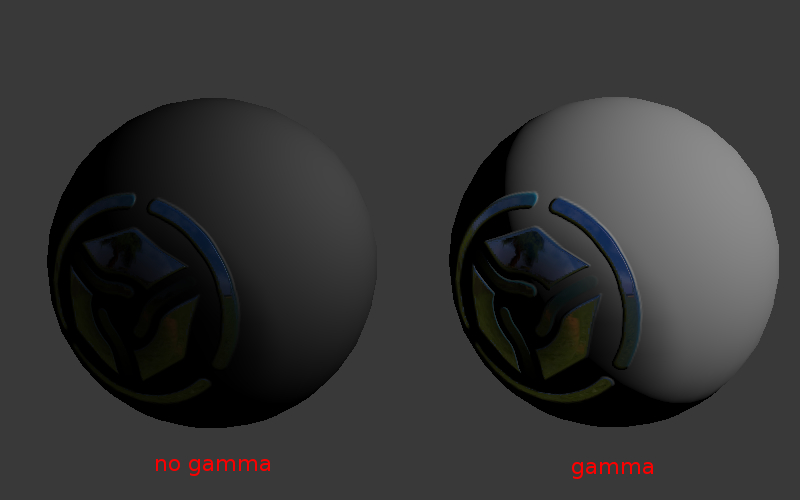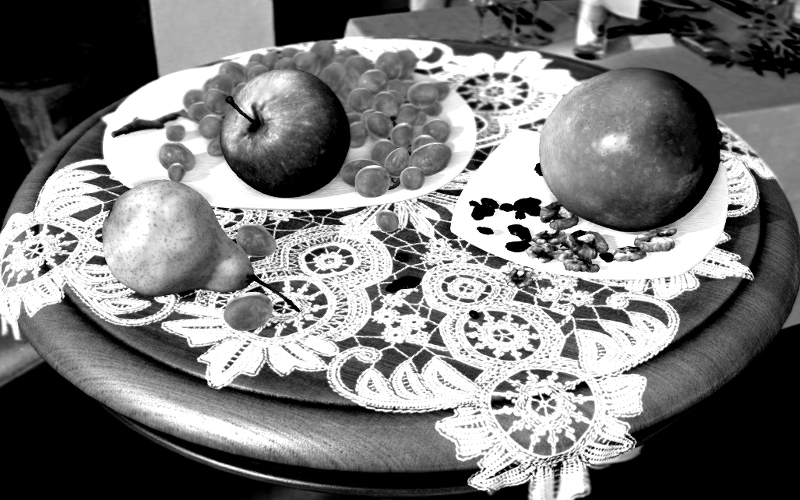色彩管理¶

Gamma概述¶

17 000

5 000

1 000

100

0.2

0.001

Color Management > Display Device > sRGB 选项为场景启用时，Blender工作于线性空间。材质的颜色和灯设置对应到相应的物理值。对于纹理图像（除法线贴图），则需要选择 Image > Input Color Space > sRGB 选项。在这种情况下，在渲染的时候执行自动图象解包（sRGB的 -> 直线）。

伽玛公式¶

Vout = Vinγ

γ<1 - 打包伽玛值，γ> 1 - 拆包伽马。在最简单的情况 1/2.2 和 2.2 的值被分别使用。在下文中，用术语 “打包” (Linear -> sRGB) 和 “解包” (sRGB -> Linear) 的术语用来代替 “伽马校正”.伽马在节点材质中¶

为遮罩的节点¶

LINEAR_TO_SRGBSRGB_TO_LINEAR 节点已被弃用。 应使用 GAMMA 节点。

GAMMA 值 γ = 0.45

GAMMA 值 γ = 0.45

alpha合成¶

概述¶

$$C_o = C_a \alpha_a + C_b \alpha_b (1 - \alpha_a)$$.

$$C_o = C_a + C_b (1 - \alpha_a)$$.

$$\alpha_o = \alpha_a + \alpha_b (1 - \alpha_a)$$.

实现¶

gl.blendFunc(gl.ONE, gl.ONE_MINUS_SRC_ALPHA);

WebGL的上下文初始化是使用 premultipliedAlpha = true 参数（即默认值）进行的。由所有颜色通道相乘 $$\alpha$$ 值在着色器进行输出。

色彩校正¶